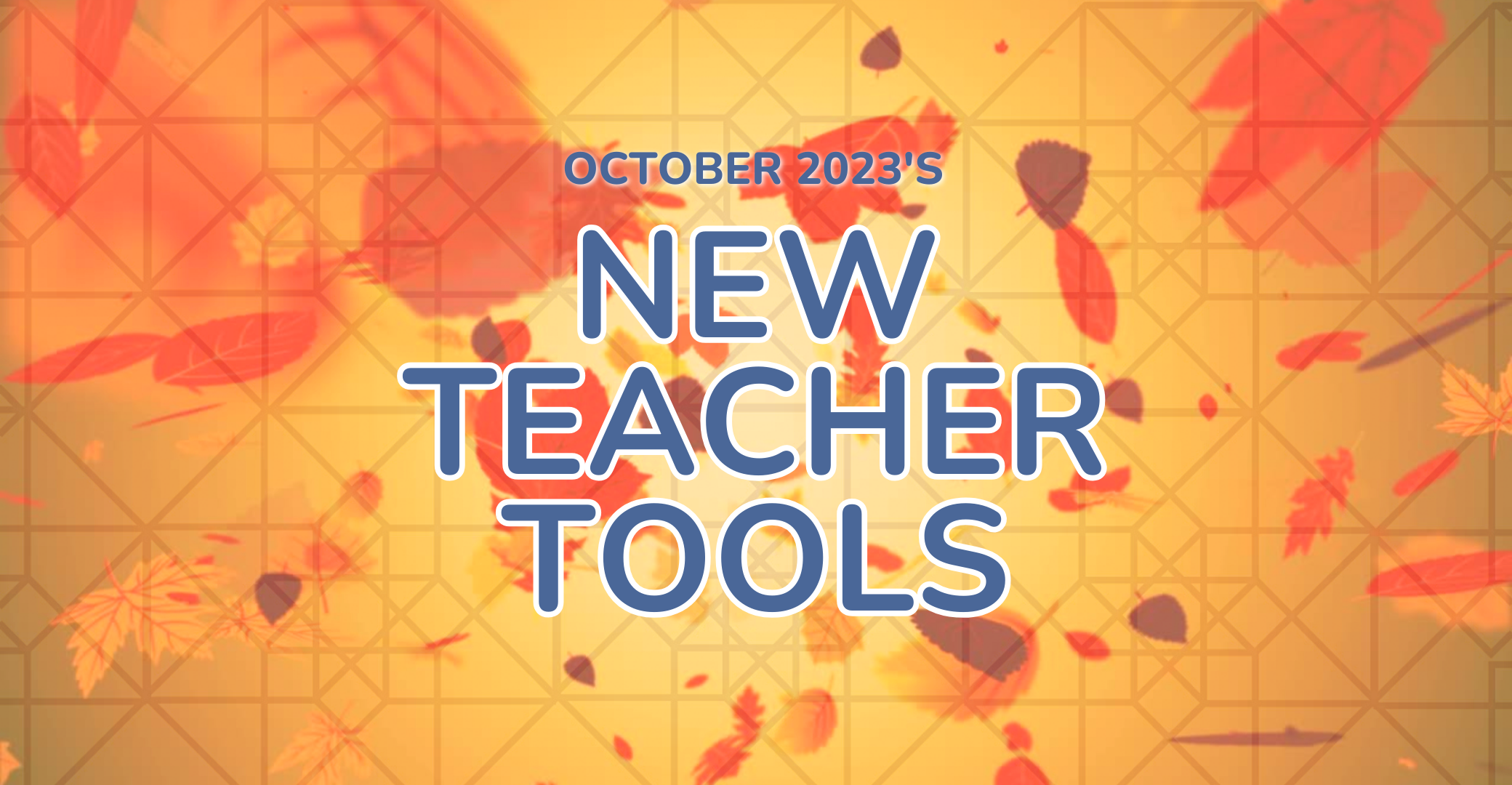# October 2023’s New Teacher Tools

Posted onHappy Fall, y’all! The weather is getting colder and the colors more spectacular, at least here in the Northeast. We have lots of new resources to share — as always, we hope they help make teaching math a little easier. (Btw, the new Geometry course and new Spanish language versions of some of our middle school materials are indicated by *** below).

• Unit 3 (Decimals) – Practice with Decimal Word Problems. This is a good set of problems that gives students practice using various operations with decimals to solve real-world problems.
• Unit 4 (Negative Numbers) – Mid-Unit Quiz – Form B
• Unit 4 (Negative Numbers) – Practice with Ordering Signed Numbers. This worksheet has students think about where various negative and positive numbers fall on number lines. Students also compare various signed numbers using greater than or less than symbols.
• ***Spanish Language Versions of the Unit 4 Mid-Unit Quiz (Form A only) and Exit Tickets
• Unit 4 (Percent) – Practice Finding Percent, Part, and Whole. In this worksheet, students get additional practice both in standard and applied problems calculating percent, finding a part, and finding a whole in the standard percentage relationship.
• Unit 4 (Percent) – Mid-Unit Quiz – Form B
• Unit 5 (Linear Expressions) – Mid-Unit Quiz – Form B
• ***Spanish Language Versions of the Unit 4 and Unit 5 Mid-Unit Quiz (Form A only) and Exit Tickets
• Unit 3 (Transformations) – Mid-Unit Quiz – Form B
• Unit 3 (Transformations) – Practice with Angles of a Triangle. This worksheet is a nice collection of numerical and algebraic problems that rely on the relationships between the interior and exterior angles of a triangle.
• ***Spanish Language Versions of the Unit 3 Mid-Unit Quiz (Form A only) and Exit Tickets.
• Unit 4 (Linear Functions) – Unit Assessment – Form B
• Unit 4 (Linear Functions) – Mid-Unit Quiz – Form A
• Unit 3 (Euclidean Triangle Geometry) – Unit Assessment – Form A
• Unit 3 (Euclidean Triangle Geometry) – Exit Tickets
• Unit 3 (Euclidean Triangle Geometry) – Triangle Inequality Exploration Activity. In this extended exploratory activity, students try to predict the number of integer lengths that the third side of a triangle can have based on two integer sides given.
• Unit 4 (Exponential and Logarithmic Functions) – General Logarithm Practice. This set of problems gives students more practice with various aspects of logarithms, including their inverse nature with exponential functions and the basic log laws.
• Unit 5 (Sequences and Series) – Arithmetic and Geometric Sequence Practice. This worksheet is a nice collection of problems that only deals with arithmetic and geometric sequences (not series). Students translate between explicit and recursive definitions of these two types of sequences as well as predicting a given term of the sequence given various pieces of information.